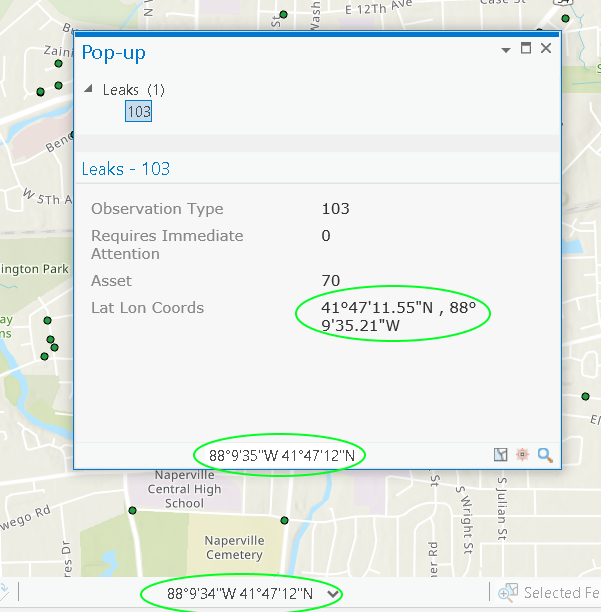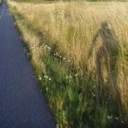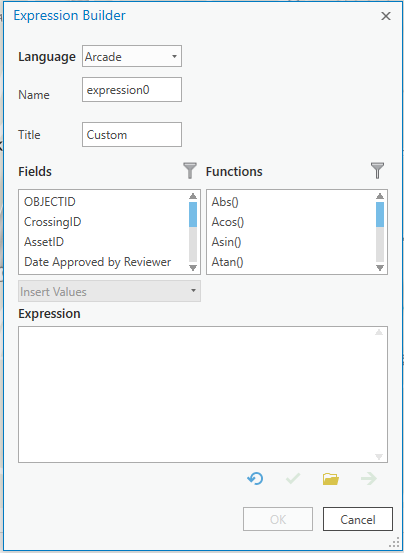display lat/long in the pop-up of a projected point feature class?

2134
6
06-27-2018 02:41 PMbyMVP

Can I use Arcade Expression or another method to create a popup in ArcGIS Pro to display the Latitude and Longitude of a point that is defined in the Web Mercator projection?

I've tried some variations of the point and geometry functions based on this reference but I have not figured out the right combination or approach.

Why would I define my data in the web mercator project you might ask?  Well that's because I'm collecting these points in the collector app and using the esri basemaps.

I don't have to use arcade if there's another method, just want to perform that spatial reference conversion to dynamically display latitude and longitude without having to add XY fields.

Kyle

1 Solution

Accepted SolutionsbyEsri Esteemed Contributor

I'm using ArcGIS Pro version 2.4.1 and the expression below works for me:

function MetersToLatLon(x, y) {
var originShift = 2.0 * PI * 6378137.0 / 2.0;
var lon = (x / originShift) * 180.0;
var lat = (y / originShift) * 180.0;
lat = 180.0 / PI * (2.0 * Atan( Exp( lat * PI / 180.0)) - PI / 2.0);
return [lat, lon];
}

function convertToDms(dd, isLng) {
var dir = "";
if (dd < 0) {
if (isLng) {
dir = "E";
} else {
dir = "W";
}
} else {
if (isLng) {
dir = "S";
} else {
dir = "N";
}
}

var absDd = Abs(dd);
var deg = Floor(absDd, 0);
var frac = absDd - deg;
var min = Floor(frac * 60, 0);
var sec = frac * 3600 - min * 60;

// Round it to 2 decimal points.
sec = Round(sec * 100) / 100;
return deg + "°" + min + "'" + sec + '"' + dir;
}

var latlon = MetersToLatLon(Geometry(\$feature).X, Geometry(\$feature).Y);

var lat = convertToDms(latlon, False);
var Lon = convertToDms(latlon, False);

return lat + " ,  " + lon;6 RepliesbyEsri Contributor

Web Mercator to LL is fortunately a simple transformation. The Arcade below transforms the geometrys coordinates from WebMercator and use the transformed values to create a URL.

//wkid 102100->4326
var g = Geometry(\$feature);
var lon = g.x/111319.49079;
var lat = g.y/6378137.0;
lat = 90*(4*Atan(Exp(Lat)) - Pi)/Pi;
return +Round(lat, 5)+","+Round(lon, 5)+"/7"byEsri Contributor

Oops, but my bad. Seems the Geometry() function is not available in Pro, only in Enterprise and AGOLbyMVP Esteemed Contributor

I must admit I haven't used popups, but the help topic...

states...

A pop-up, by default, shows a listing, grouped by layer, of all identified items and the attributes of one feature at a time. The attribute portion allows you the flexibility to view configured content (custom text, field-value pairs, charts, images, and so on).

Which suggests that perhaps having the data already in a text field as a concatenation of a Longitude-Latitude field might be one route.byMVP

My data is a hosted feature service for the collector app.  I don't want to have to calculate attribtues in the hosted feature service layer each time a point is added or moved or set up some nonsense CDC process to do this.

I dont actually need the lat/long in the popup, what I really need is the lat/long as URL tokens behind an image to link to google maps, bing maps, and most of all, mapillary at a particular location and scale.  If I could get the coordinates in the popup I can get them in a url expression I think, but the popup would be an easy test to see that yes, it can be done.

I will look at the math solution there Håkon Dreyer maybe I can get that to work with the point[() function?  That is nice to know, I figured a mathematical projection calculation might be part of a solution.

Maybe I will take this to the Ideas page.byMVP

In the popup configuratior  for the latest version of pro, there is an option to add text to the popup, then at the bottom of the configurator, a button for expressions. Click that button, then there is another button that says "new".  Click NEW then we get this window:In this expression builder, there are only text, number, and date functions.  also in this expression builder, the SHAPE field is not presented as an actionable field.

So, looks like a "cant be done" for now in pro.  To the ideas page!byEsri Esteemed Contributor

I'm using ArcGIS Pro version 2.4.1 and the expression below works for me:

function MetersToLatLon(x, y) {
var originShift = 2.0 * PI * 6378137.0 / 2.0;
var lon = (x / originShift) * 180.0;
var lat = (y / originShift) * 180.0;
lat = 180.0 / PI * (2.0 * Atan( Exp( lat * PI / 180.0)) - PI / 2.0);
return [lat, lon];
}

function convertToDms(dd, isLng) {
var dir = "";
if (dd < 0) {
if (isLng) {
dir = "E";
} else {
dir = "W";
}
} else {
if (isLng) {
dir = "S";
} else {
dir = "N";
}
}

var absDd = Abs(dd);
var deg = Floor(absDd, 0);
var frac = absDd - deg;
var min = Floor(frac * 60, 0);
var sec = frac * 3600 - min * 60;

// Round it to 2 decimal points.
sec = Round(sec * 100) / 100;
return deg + "°" + min + "'" + sec + '"' + dir;
}

var latlon = MetersToLatLon(Geometry(\$feature).X, Geometry(\$feature).Y);

var lat = convertToDms(latlon, False);
var Lon = convertToDms(latlon, False);

return lat + " ,  " + lon;